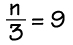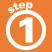Home    |    Teacher    |    Parents    |    Glossary    |    About UsTo solve a division equation, use the inverse operation of multiplication. Multiply both sides by the same number.

Click the equation to see how to solve it.Homework Help | Algebra | Equations and InequalitiesEmail this page to a friendSearch·  Solving addition and     subtraction equations·  Multiplication equations·  Division equations·  Inequalities·  Formulas·  Two-step equations     and inequalitiesFirst Glance In Depth Examples WorkoutSolving division equations